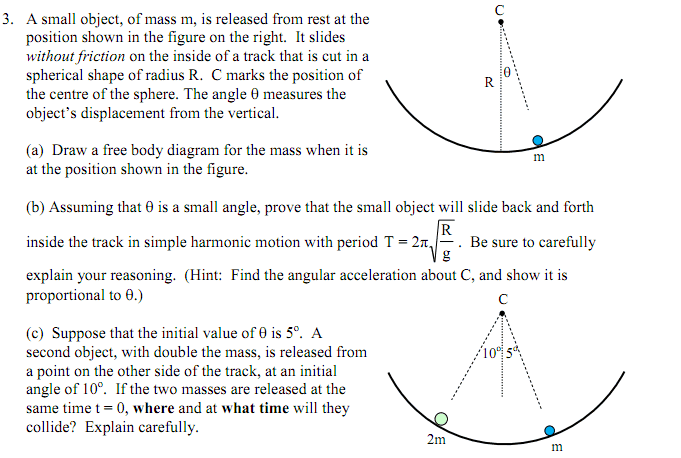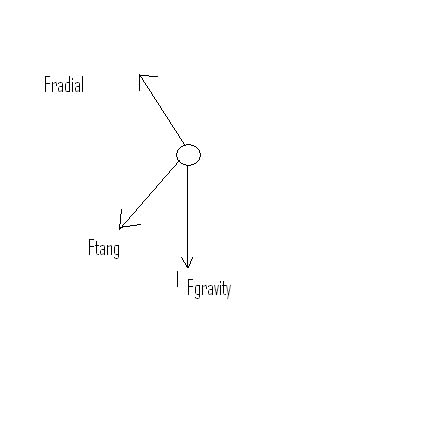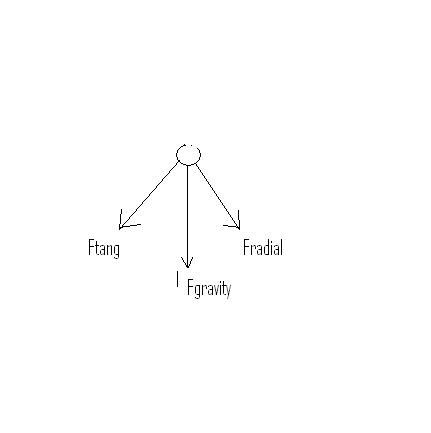# Simple Harmonic Motion Problem - Need help! Thanks

## Homework Statement

Below is the image of the question I am having trouble with.

B) and C) I need help with!## The Attempt at a Solution

Can anyone point me in the right direction? B and C are my trouble questions.

I dont know how to prove that the small object will slide back and forth
inside the track in simple harmonic motion with period

T = 2π sqrt (r/g)

## Answers and Replies

Delphi51
Homework Helper
Wow, interesting question. It was a thrill to do it. I certainly don't want to spoil it for you, but will help if you try. How will you go about finding the angular acceleration? Can you find the force on the mass and use F = ma?

I rather think you will need to know how to do derivatives to complete it.

Curious3141
Homework Helper
Part b) is simple. No need for calculus. All you need to do is to identify the forces acting on the mass. The component of force tangential to the surface (which is solely due to the weight and not contributed to by the normal force) is responsible for the tangential acceleration. Express tangential acceleration in terms of R and $\ddot{\theta}$ (latter is the angular acceleration).

It's a simple trig equation, which you can render into a simple algebraic equation by applying a well-known small angle approximation. You can then show that angular acceleration is proportional to the angle for small angles, and this is the condition for SHM. Then compare this to the standard form for SHM, equate the correct term to $\omega^2$, do some rearrangement to find the period T, et voila!

Curious3141
Homework Helper
c) is also easy (almost trivially easy when you realise the answer). Think about this: are the periods of the two masses the same? How long does it take each of them to reach their equilibrium positions? Do their equilibrium positions coincide?

Last edited:
Have you had to do a similar exercise for a simple pendulum?
Do you see any similarity?

Wow, interesting question. It was a thrill to do it. I certainly don't want to spoil it for you, but will help if you try. How will you go about finding the angular acceleration? Can you find the force on the mass and use F = ma?

I rather think you will need to know how to do derivatives to complete it.

I would really appreciat eyou helping me out through this. I really want to learn how to do this PROPERLY!

I am not 100% sure how I would go about finding the angular acceleration?

Delphi51
Homework Helper
Start with the force on the mass. Figure out what acceleration it causes. Then convert to rotational acceleration and follow the hint in the question.

Im still having issues with b . What is my first step?

Delphi51
Homework Helper
What do you have for your F = ma statement?
From that, you can use Curious3141's idea of comparing it with the equation of motion for simple harmonic motion. Or you could use calculus to find a solution and actually see the kind of motion it is for yourself.

Show us what you have for F = ma and we can help you with the next step. If you aren't sure you have that right, it would save time if you showed your result from (a). You could sketch it in a paint program to make it easy to show here.

I am not really sure how the f=ma part

Attached is my FBDDelphi51
Homework Helper
Pretty good! The F radial should go the opposite way, where it is cancelled out by the force of the track on the mass. Now you need to get the angle theta on your diagram and get an expression for Ftang in terms of the angle, mass and g. Finally, say that force equals ma.

So this is correct (image)So, Fradial cancels out - Ok!

Dont quite understand what you said here :( "Now you need to get the angle theta on your diagram and get an expression for Ftang in terms of the angle, mass and g. Finally, say that force equals ma."

Delphi51
Homework Helper
Extend your diagram to include the diagram given in the question with the θ on it.
Figure out where θ goes on your part. Is it between Ftang and Fgravity or what?
You must find the angle between Ftang and Fgravity - it might be θ or something like 90 - θ. Then make a right triangle with hypotenuse Fgravity, side Ftang and one more side you add at 90 degrees to Ftang. Use trigonometry to express the relation between Ftang and Fgravity.